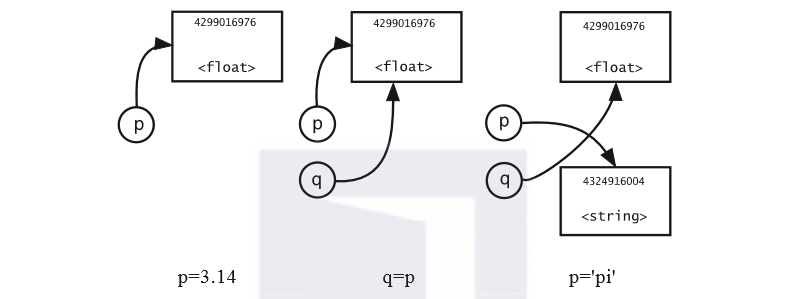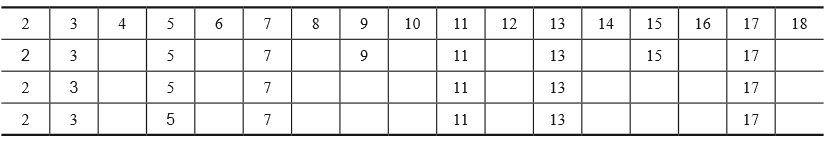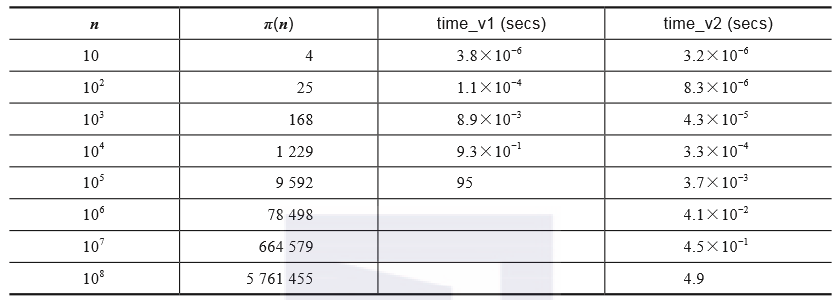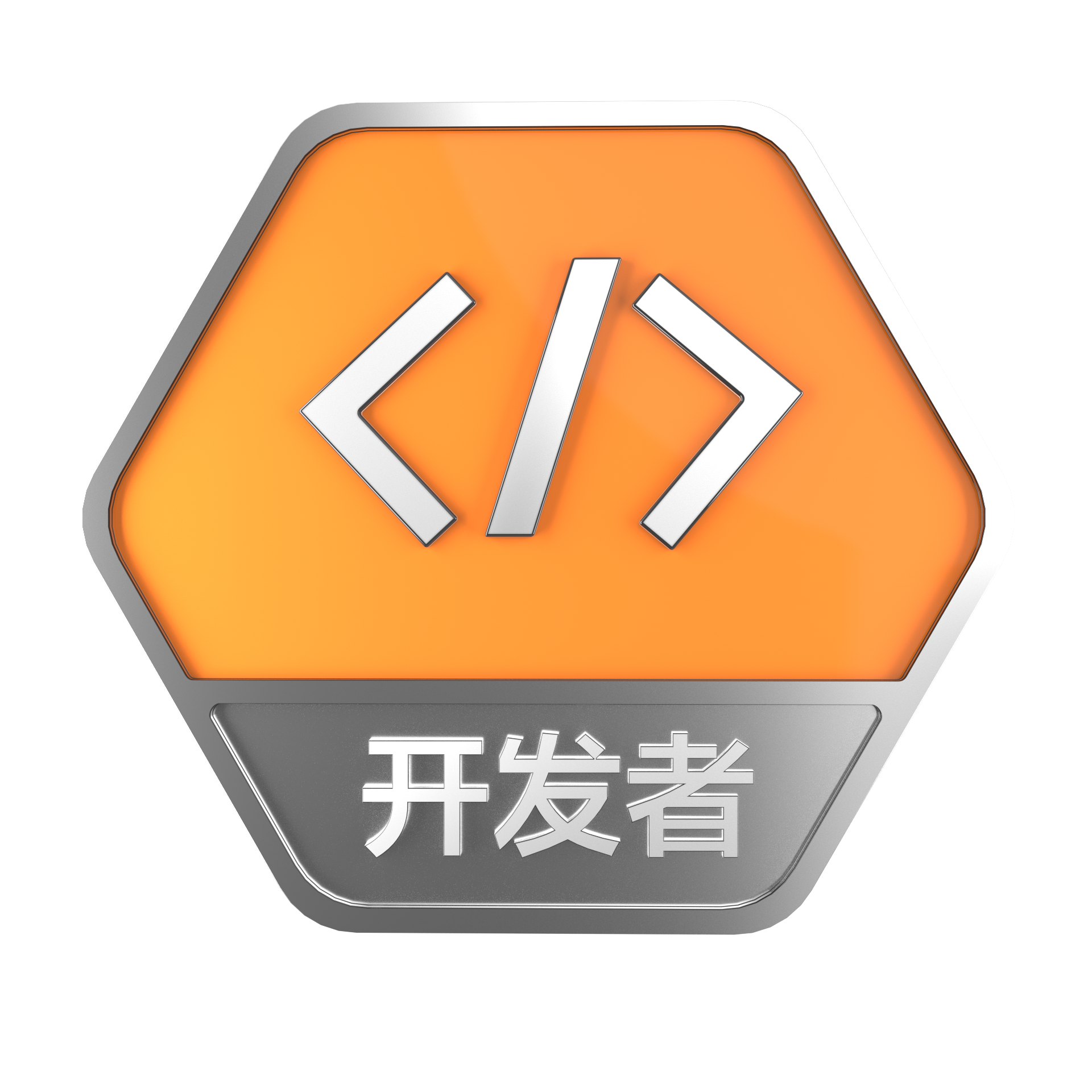带你读《Python科学计算（原书第2版）》之三：Python简明教程

+关注继续查看

|第3章|
Python for Scientists, Second Edition

3.1　输入Python代码

Python允许两种形式的注释（comment）。一种是#符号，表示当前行中#符号后的其余部分是注释。文档字符串（docstring）可以跨越多行，并且可以包含任何可打印字符。文档字符串由一对三引号来界定，例如：
""" This is a very short docstring. """

a=4; b=5.5; c=1.5+2j; d='a'
e=6.0*a-b*b+\
c**(a+b+c)
f=6.0*a-b*b+c**(
a+b+c)
a, b, c, d, e, f

3.2　对象和标识符

Python包含大量的对象和标识符。对象可以被认为是一个计算机内存区域，包含某种数据以及与数据相关的信息。对于一个简单的对象，这些信息包括它的类型和标识（即内存中的位置，很显然这与计算机相关）。因此，大多数用户对对象标识并不感兴趣。用户需要与机器无关的访问对象的方法，这可以通过标识符提供。标识符是附加到对象上的一个标签，由一个或者多个字符组成。标识符的第一个字母必须是字母或者下划线，后续字符必须是数字、字母或者下划线。标识符是区分大小写的：x和X是不同的标识符。（以下划线开始或结束的标识符具有专门用途，因此初学者应该避免使用。）我们必须避免使用预定义的标识符（如list），并且应该总是尝试使用有意义的标识符。然而，选择xnew、x_new或xNew，则是用户个人的偏好。请尝试运行如下代码片段，建议读者在终端窗口中逐行键入，可以更加明确其含义：

1 p=3.14
2 p
3 q=p
4 p='pi'
5 p
6 q=

3.3　数值类型

Python包括三种简单的数值对象类型，我们还将介绍第四种类型（稍微复杂）。

3.3.1　整型

Python语言中整型数据为int。早期版本支持的整型数值范围为[-231, 231-1]，但是新的版本整型数值范围进一步扩大，现在Python支持的整型数值范围几乎没有限制（仅受计算机内存限制）。

p=mq+n，其中0≤n＜q

3.3.2　实数

Python语言中浮点数为float。在大多数安装环境中，浮点数的精度大约为16位，其数值范围为(10-308, 10308)。浮点数对应于C语言家族中的double，对应于Fortran家族中的real*8。浮点数常量的标记遵循一般标准，例如：
-3.14, -314e-2, -314.0e-2, -0.00314E3

Python语言具有一个继承于C语言的有用特性。假设我们希望将a引用的浮点数递增2，很显然可以使用如下代码：

temp=a+2
a=temp

a+=2

x=m+y，其中0≤y＜1.0

import math
dir(math)        # or math.<TAB> in IPython

math.atan2? # or help(math.atan2)

from math import *

from cmath import *

3.3.3　布尔值

int和float类型值（如x、y）定义了标准的相等运算符，例如x==y（等于）、x!=y（不等于）。为了提醒读者注意Python浮点数的局限性，下面是一个简单的练习，请猜测以下代码行的运行结果，然后键入、运行该行代码并解释其执行结果。
math.tan(math.pi/4.0)==1.0

(0<=x) and (x<1) and (1z)

3.3.4　复数

Python复数支持五种基本的算术运算，并且在混合运算模式中，将自动进行向上数值类型转换。另外，还包含一个针对复数运算的数学函数库，这需要导入库cmath，而不是math。然而，根据显而易见的原因，复数没有定义前文描述的涉及排序的比较运算，但可以使用等于运算符以及不等于运算符。

3.4　名称空间和模块

Python的优势之一是它能够包含由读者或者其他人编写的文件（其中包含对象、函数等）。为了实现这种包含其他文件的功能，假设读者已经创建了一个包含可以重用的对象（如obj1和obj2）的文件，并且保存了文件（例如，保存为foo.py，其后缀必须为.py。请注意，对于大多数文本编辑器而言，都要求该文件后缀为.py，以便支持处理Python代码）。这种Python文件被称为模块（module）。模块（foo.py）的标识符为foo，即其文件主名，不包括其后缀。

import foo
（当首次导入该模块时，会将其编译成字节码并写入磁盘文件foo.pyc。在随后的导入中，解释器直接加载这个预编译的字节码，除非foo.py的修改日期更近，在这种情况下，将自动生成文件foo.pyc的新版本。）

from foo import obj1, obj2

from foo import *

from math import *

from cmath import *

import math as re
import cmath as co

if name == "__main__"

3.5　容器对象

3.5.1　列表

1 [1,4.0,'a']
2 u=[1,4.0,'a']
3 v=[3.14,2.78,u,42]
4 v
5 len(v)
6 len?        # or help(len)
7 v*2
8 v+u
9 v.append('foo')
10 v

3.5.2　列表索引3.5.3　列表切片

1 u=[0,1,2,3,4,5,6,7]
2 su=u[2:4]
3 su
4 su=17
5 su
6 u
7 u[2:4]=[3.14,'a']
8 u

3.5.4　列表的可变性

1 a=4
2 b=a
3 b='foo'
4 a
5 b
6 u=[0,1,4,9,16]
7 v=u
8 v='foo'
9 v
10 u

1 u=[0,1,4,9,16]
2 v=u[ : ]
3 v='foo'
4 v
5 u

3.5.5　元组

(a,b,c,d)=(4,5.0,1.5+2j,'a')
a,b,c,d = 4,5.0,1.5+2j,'a'

temp=a
a=L1
L1=temp

a,L1 = L1,a

3.5.6　字符串

s1="It's time to go"
s2=' "Bravo!" he shouted.'

L = [1,2,3,5,8,13]
ls = str(L)
ls
eval(ls) == L

3.5.7　字典

1 empty={}
2 parms={'alpha':1.3,'beta':2.74}
3 #parms=dict(alpha=1.3,beta=2.74)
4 parms['gamma']=0.999
5 parms

3.6　Python的if语句

if <布尔表达式>:

<代码块1>

<代码块2>
<布尔表达式>的求值结果必须为True或者False。如果为True，则执行<代码块1>，然后执行<代码块2>（程序的剩余部分）；如果<布尔表达式>为False，则仅执行
<代码块2>。注意，if语句以冒号（:）结束，这表明必须紧跟一个代码块。不使用括号分隔符的优点是逻辑更简单，但缺点是必须仔细保证缩进的一致性。所有支持Python语言的编辑器都会自动进行处理。

x=0.47
if 0<x<1:
print "x lies between zero and one."
y=4

if语句的简单通用语法格式如下：
if <布尔表达式>:

<代码块1>

else:

<代码块2>

<代码块3>

if <布尔表达式1>:

<代码块1>

elif <布尔表达式2>:

<代码块2>

elif <布尔表达式3>:

pass

else:

<代码块4>

<代码块5>

if x>=0:

y=f

else:

y=g

y=f if x>=0 else g

3.7　循环结构

3.7.1　Python的for循环结构

for <迭代变量> in <可迭代对象>:

<代码块>

c=4
for c in "Python":
print c

for (x,y) in Z:

<代码块>

range(start,end,step)
range函数生成一个整数列表：[start,start+step,start+2*step,...]，每个整数都小于end。（我们在3.5.3节曾讨论过这个概念。）此处，step是可选参数，其缺省值为1；start也是可选参数，其缺省值为0。因此range(4)的结果为[0,1,2,3]。请阅读如下代码：

L=[1,4,9,16,25,36]
for it in range(len(L)):
L[it]+=1
L

for it in range(4):
it*=2
print it
it

3.7.2　Python的continue语句

for <迭代变量> in <可迭代对象>:

<代码块1>
if <测试1>:
continue
<代码块2>

<代码块5>

3.7.3　Python的break语句

break语句允许中断循环，也可以通过使用一个else子句，得到不同的结果。其基本语法格式如下：
for <迭代变量> in <可迭代对象>:

<代码块1>
if <测试2>:
break
<代码块2>

else:

<代码块4>

<代码块5>

y=107
for x in range(2,y):

if y%x == 0:
print y, " has a factor ", x
break

else:

print y, " is prime."

3.7.4　列表解析

L1=[2,3,5,7,11,14]
L2=[]        # Empty list
for i in range(len(L1)):
L2.append(L1[i]**2)
L2

L1=[2,3,5,7,11,14]
L2=[x**2 for x in L1]
L2

L2=[x*x for x in L1 if x%2]

import math
lpoints=[(1,0),(1,1),(4,3),(5,12)]
ldists=[math.sqrt(x*x+y*y) for (x,y) in lpoints]

l_x=[0,2,3,4]
l_y=[1,2]
l_dist=[math.sqrt(x*x+y*y) for x in l_x for y in l_y]

3.7.5　Python的while循环

Python语言支持的另一种非常有用的循环结构是while循环。其最简单的语法格式如下：
while <测试表达式>:

<代码块1>

<代码块2>

while True :
print "Type Control-C to stop this!"

3.8　函数

3.8.1　语法和作用范围

Python函数可以定义在程序的任何地方，但必须是在实际使用之前。其基本语法如下面的伪代码所示：
def <函数名称>(<形参列表>):

<函数体>

2    """ Takes x and returns x+1. """
3    x = x+1
4    return x
5
6 x=23
9 x

2    """ Adds y to x and returns x+y. """
3    return x+y
4

y=1

2    """ Add x and y and return them and their sum. """
3    z=x+y
4    return x,y,z
5
6 a, b, c = add_x_and_y(1,0.456)
7 a, b, c

f(0.456, 1)
f

1 L = [0,1,2]
2 print id(L)
4    """ Increment first element of list. """
5    M+=1
6
8 print L
9 id(L)

1 L = [0,1,2]
2 id(L)
4    """ Increment first element of list. """
5    MC=M[ : ]
6    MC+=1
7    return MC
8
10 L
11 id(L)

def foo1(a,b,c):

<函数体>

3.8.3　关键字参数

def foo2(d=21.2, e=4, f='a'):

<代码块>

def foo3(a,b,c,d=21.2,e=4,f='a')

<代码块>

3.8.4　可变数量的位置参数

1 def average(*args):
2    """ Return mean of a non-empty tuple of numbers. """
3    print args
4    sum=0.0
5    for x in args:
6        sum+=x
7    return sum/len(args)
8
9 print average(1,2,3,4)
10 print average(1,2,3,4,5)

3.8.5　可变数量的关键字参数

Python可以完美处理下列形式的函数：该函数接受固定数量的位置参数；随后是任意数量的位置参数；再随后是任意数量的关键字参数—因为必须遵守“位置参数位于关键字参数之前”的顺序规则。如前一节所述，附加的位置参数被打包成元组（由星号标识）。附加的关键字参数则被封装到字典中（由两个星号标识）。如下示例说明了这个过程：

1 def show(a, b, *args, **kwargs):
2    print a, b, args, kwargs
3
4 show(1.3,2.7,3,'a',4.2,alpha=0.99,gamma=5.67)

3.8.6　Python的输入/输出函数

name = raw_input("What is your name? ")
print "Your name is " + name

ilist = input('Enter an explicit list')
ilist

3.8.7　Python的print函数

print <要打印输出的内容>

Print(<要打印输出的内容>)

from future import print_function

print命令要求使用一个元组作为参数。因此，假设it指向一个整数，y指向一个浮点数，不使用元组分隔符（括号），则可以编写如下语句：

print "After iteration ",it,", the solution was ",y

print "After iteration %d, the solution was %f" % (it,y)

%

%-10.3f的输出结果相同，只是左对齐而已。与整数格式化一样，紧跟在百分号后面的正号强制输出结果带正号或者负号。另外，%010.3f会把前面的空白符替换为0。

%-13.4e的输出结果为左对齐，%+13.4e输出结果的前面包含一个正号和2个空白符。（%+-13.4e结果相同但是左对齐。）如果宽度少于最低10个的要求，则Python会把宽度增加到10。最后，在格式化字符串代码中把'e'替换为'E'，则结果使用大写的字母E，例如%+-13.4E的输出结果为“+1.2346E+06”。

3.8.8　匿名函数

f= lambda x, y : x, y, x+y

lambda x,y:x,y,x+y

3.9　Python类简介

1 # File frac.py
2
3 import gcd
4
5 class Frac:
6    """ Fractional class. A Frac is a pair of integers num, den
7    (with den!=0) whose GCD is 1.
8    """
9
10    def __init__(self,n,d):
11        """ Construct a Frac from integers n and d.
12            Needs error message if d=0!
13        """
14        hcf=gcd.gcd(n, d)
15        self.num, self.den = n/hcf, d/hcf
16
17    def __str__(self):
18        """ Generate a string representation of a Frac. """
19        return "%d/%d" % (self.num,self.den)
20
21    def __mul__(self,another):
22        """ Multiply two Fracs to produce a Frac. """
23        return Frac(self.num*another.num, self.den*another.den)
24
26        """ Add two Fracs to produce a Frac. """
27        return Frac(self.num*another.den+self.den*another.num,
28                  self.den*another.den)
29
30    def to_real(self):
31        """ Return floating point value of Frac. """
32        return float(self.num)/float(self.den)
33
34 if __name__=="__main__":
35    a=Frac(3,7)
36    b=Frac(24,56)
37    print "a.num= ",a.num, ", b.den= ",b.den
38    print a
39    print b
40    print "floating point value of a is ", a.to_real()
41    print "product= ",a*b,", sum= ",a+b1.分别创建名为__div__和__sub__的除法和减法类函数，并测试。
2.如果分母为1，则打印输出的结果会显得有些奇怪，例如7/1。请改进__str__函数，使得当self.den为1时，创建的字符串恰好是self.num，并测试新版本是否正常工作。
3.当参数d为0时，很显然__init__会出错。请为用户提供警告信息。

3.11　素数：实用示例1 def sieve_v1(n):
2    """
3        Use Sieve of Eratosthenes to compute list of primes <= n.
4        Version 1
5    """
6    primes=range(2,n+1)
7    for p in primes:
8        if p*p>n:
9            break
10        product=2*p
11        while product<=n:
12            if product in primes:
13                primes.remove(product)
14            product+=p
15    return len(primes),primes

from sieves import sieve_v1
sieve_v1?
sieve_v1(18)

timeit sieve_v1(1000)1 def sieve_v2(n):
2    """
3        Sieve of Eratosthenes to compute list of primes <= n.
4        Version 2.
5    """
6    sieve = [True]*(n+1)
7    for i in xrange(3,n+1,2):
8        if i*i > n:
9            break
10        if sieve[i]:
11            sieve[i*i: :2*i]=[False]*((n - i*i) // (2*i) + 1)
12    answer =  + [i for i in xrange(3,n+1,2) if sieve[i]]python实现身份证校验位计算

1042 0PAI-AutoLearning 图像分类使用教程
PAI AutoLearning（简称PAI AL）自动学习支持在线标注、自动模型训练、超参优化以及模型评估。在平台上只需准备少量标注数据，设置训练时长即可得到深度优化的模型。同时自动学习PAI AL平台与EAS模型在线服务打通，一键完成模型部署。下面通过一个番茄(tomato)和黄瓜(cucumber)的图片分类示例来演示整个流程的实现具体操作实现步骤。
647 03757 0931 03912 0java的nio之：java的nio系列教程之SocketChannel
Java NIO中的SocketChannel是一个连接到TCP网络套接字的通道。可以通过以下2种方式创建SocketChannel： 打开一个SocketChannel并连接到互联网上的某台服务器。
912 0《Python算法教程》——第2章 基础知识 2.1 计算领域中一些核心理念
1594 0A Byte of Python(简明Python教程) for Python 3.0 下载
A Byte of Python v1.92 (for Python 3.0) 官方下载地址，当前(20120730)  最新版本 1.92 基于Python3的 下载： http://files.swaroopch.com/python/byte_of_python_v192.pdf在线阅读：http://www.cnblogs.com/txw1958/archive/2012/12/10/A_Byte_of_Python3.html 简明Python3教程《A Byte of Python3》是一本关于用Python3语言编程的书。
2042 0java的nio之：java的nio系列教程之selector

924 0+关注
560

1795

+ 订阅

《2021云上架构与运维峰会演讲合集》

《零基础CSS入门教程》

《零基础HTML入门教程》